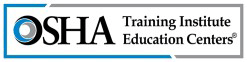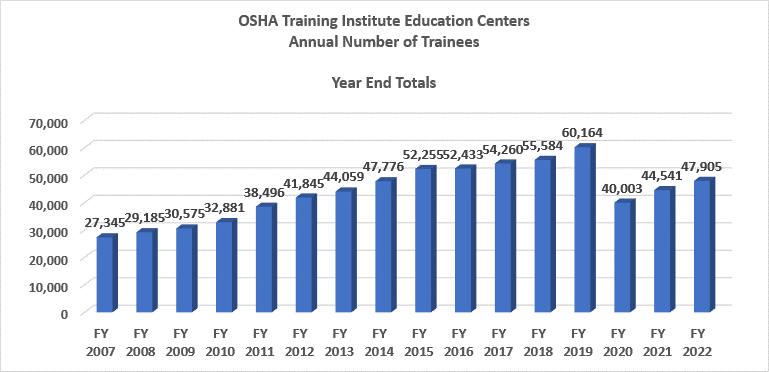### Program Statistics#### Number of trainees in the last 5 years = 248,197

Text version of chart:

Title:
OTI Education Centers Annual Number Trained
Chart Type:
Vertical Bar Graph
Graph Elements:
16 – one bar for each fiscal year between 2007 and 2022

Values:

• FY 2007 = 27,345
• FY 2008 = 29,185
• FY 2009 = 30,575
• FY 2010 = 32,881
• FY 2011 = 38,496
• FY 2012 = 41,845
• FY 2013 = 44,059
• FY 2014 = 47,776
• FY 2015 = 52,255
• FY 2016 = 52,433
• FY 2017 = 54,260
• FY 2018 = 55,584
• FY 2019 = 60,164
• FY 2020 = 40,003
• FY 2021 = 44,541
• FY 2022 = 47,905